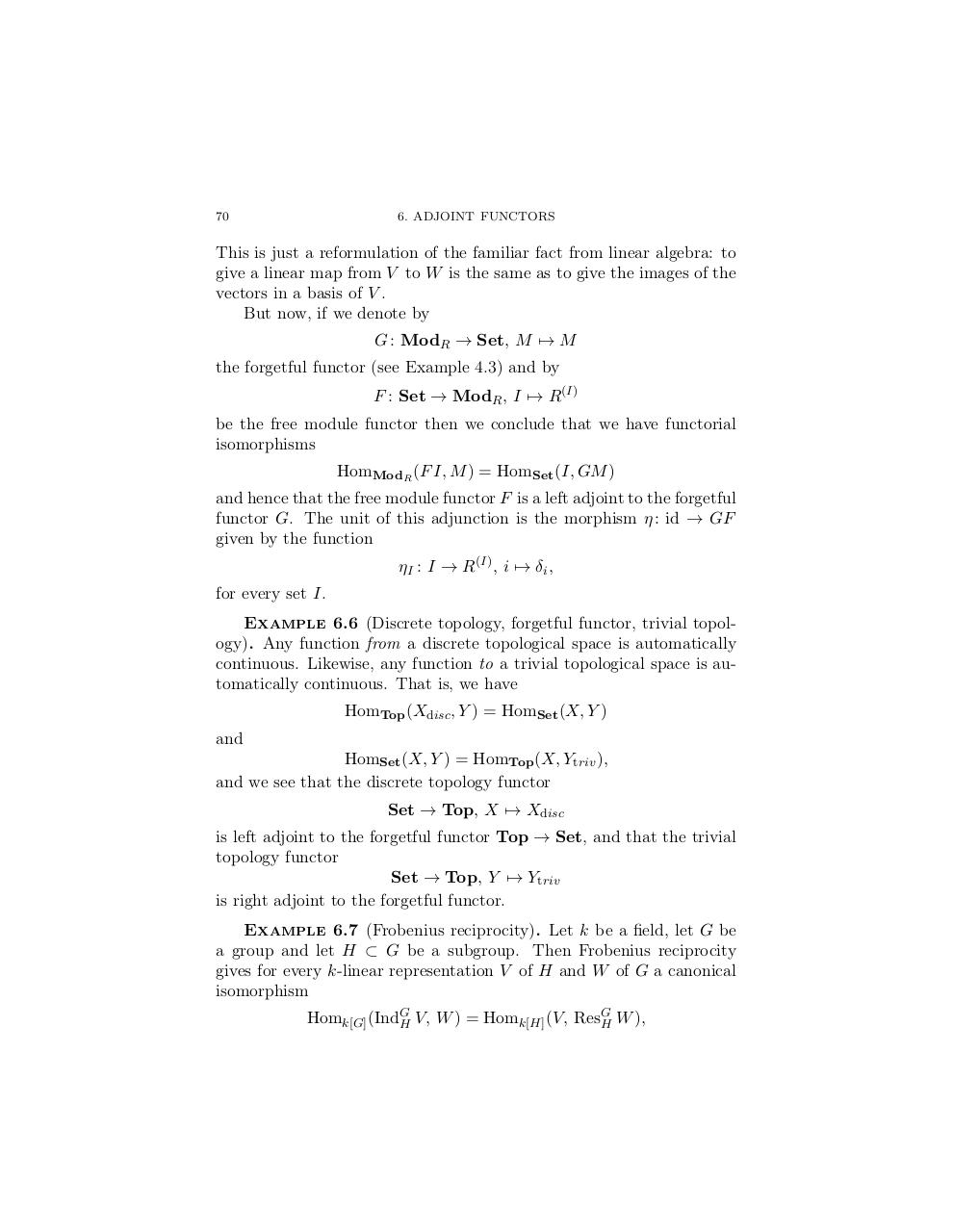# yoneda lenny.pdfPage 1 2 3 4 5 6 7 8 9

#### Text preview

70

This is just a reformulation of the familiar fact from linear algebra: to
give a linear map from V to W is the same as to give the images of the
vectors in a basis of V .
But now, if we denote by
G : ModR → Set, M 7→ M
the forgetful functor (see Example 4.3) and by
F : Set → ModR , I 7→ R(I)
be the free module functor then we conclude that we have functorial
isomorphisms
HomModR (F I, M ) = HomSet (I, GM )
and hence that the free module functor F is a left adjoint to the forgetful
functor G. The unit of this adjunction is the morphism η : id → GF
given by the function
ηI : I → R(I) , i 7→ δi ,
for every set I.
Example 6.6 (Discrete topology, forgetful functor, trivial topology). Any function from a discrete topological space is automatically
continuous. Likewise, any function to a trivial topological space is automatically continuous. That is, we have
HomTop (Xdisc , Y ) = HomSet (X, Y )
and
HomSet (X, Y ) = HomTop (X, Ytriv ),
and we see that the discrete topology functor
Set → Top, X 7→ Xdisc
is left adjoint to the forgetful functor Top → Set, and that the trivial
topology functor
Set → Top, Y 7→ Ytriv
is right adjoint to the forgetful functor.
Example 6.7 (Frobenius reciprocity). Let k be a field, let G be
a group and let H ⊂ G be a subgroup. Then Frobenius reciprocity
gives for every k-linear representation V of H and W of G a canonical
isomorphism
G
Homk[G] (IndG
H V, W ) = Homk[H] (V, ResH W ),